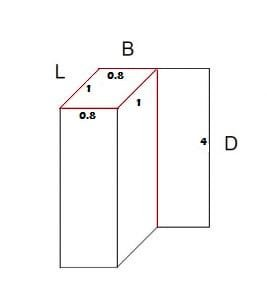## How To Calculate Area Shuttering:

Shuttering or formwork is a temporary, rigid structure in which the cast in situ concrete is poured for casting the members to required shape. Different formworks are used for different shape members.

In this article, we will discuss how to calculate the shuttering area before construction.

Before calculating the shattering area first, we need to know how to calculate the peripheral length of any shape.

## Peripheral Length/Perimeter:

We know that perimeter is the distance around a 2D (two dimensional) shape.

For example, square has four sides. Let ‘s’ be the length of one side, then

The peripheral length = s+s+s+s = 4s

Rectangle has four sides also but the length and breadth is different, let one side length is ‘l’ and breadth is ‘b’

Peripheral length = l+b+l+b = 2l+2b

Now coming to the calculation of shuttering area.

Shttering area = Pheripheral length * Depth

Let me give you an examplePheripheral length = (2 * 1) + (2*0.8) = 2 +1.6 =3.6

Total shuttering area = 3.6 * 4 = 14.4 sq.m